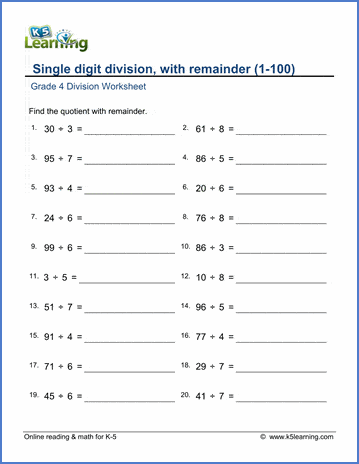# Grade 4 Mental Division Worksheets

## Division worksheets

These grade 4 worksheets provide practice in division problems which can be attempted without extensive calculation so as to emphasize the concept and notation of division rather than calculations. Some questions involve remainders.

## Mental Division

Division facts practice (1-10) 9 ÷ 3 =
Division facts practice (1-12) 88 ÷ 8 =
Division facts (missing dividend or divisor) 63 ÷ ___ = 9

Divide by 10 or 100 900 ÷ 10 =
Divide by whole tens or hundreds 900 ÷ 30 =
Divide whole tens or hundreds by 1-digit 280 ÷ 4 =

Single digit division (no remainder) 728 ÷ 4 =
Division with remainder (1-100) 69 ÷ 4 =

Division with remainder, divisor a whole ten 91 ÷ 10 =
Division with remainder, divisor a whole hundred 910 ÷ 100

## Division word problems

Multiplication and division word problems Word problems
Mixed 4 operations word problems Word problems